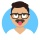Home
IT Knowledge
Inspiration
Languages
EN

# Python - parse string to float

0 points
Created by:Zachary
316

In this article, we would like to show you how to parse the string to float in Python.

Quick solution:

``````text = "10.99"
print(float(text2))  # 10.99``````

## Practical example

In this example, we present how to parse the string to a float.

``````text1 = "10"
text2 = "10.99"

print(float(text1))  # 10.0
print(float(text2))  # 10.99``````

Output:

``````10.0
10.99``````